## Mathematics of Weighted Polynomial Regression

Weighted polynomial regression is a method by which some terms are considered more strongly than others. There are a number of reasons this may be desired. If the regression coefficients are being used to extrapolate additional data it may make for a more accurate prediction if the newest data is considered more strongly than older data. A set of data that is based on measurements might have a margin of error that is unique for each data point. The data points with lower margins of error can be considered more strongly than those of higher error. A set of data points might also represent an average over a different amount of data. In this case, the amount of data each point represents can be used as the weight. There are a number of reasons to weight data points individually.

We will begin with an example of a weighted average. A classic example has to do with considering an average of averages, where each average has a different number of data points. Consider two classes that have taken the same test. One class has 20 students, the other 30.

First class:

 71 71 68 68 67 67 70 73 73 65 72 67 68 70 69 74 72 67 74 73

Second class:

 94 85 86 89 91 94 86 92 89 93 91 89 89 88 94 92 86 91 85 92 91 92 89 85 91 94 94 91 91 86

The average for the first class is 70.0, and for the second class 90.0. The average of all the grades together is 82.0. However, the average of the two averages is 80.0. This is because the average of averages considers both sets to be equal. This can be corrected by considering the number of students.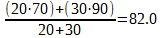Here, a weighting value has been added to each term and reproduces the same value as the average of all data points. Let us look at this in a general form: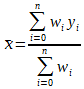To prove this, let's plug in the values:

 w0 20 w1 30 y0 70 y1 90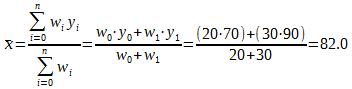To see how we came to the general form, let us consider how to introduce a weighting term into the average. The mean average is actually just polynomial regression of degree 0. So let's write out the average as polynomial regression in expanded matrix form: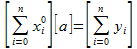This doesn't look like the mean average, but it can be show to be so if we reduce. Here a is the average. A 1x1 matrix is the same as if it wasn't in a matrix, so we can remove the matrices.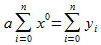Normally one does not see that averages have x values associated with y values, but they do—kind of. The reason they are not seen is because the x values are raised to the 0th power, which means every x value is just 1. And the summation of a sequence of 1 is just the count of that sequence (if you add up n ones, you get n). So the series can be reduced: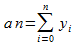Now solve for a by moving n to the opposite side: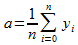And this is how one is use to seeing the average represented. Thus our matrix representation is equivalent. Let's return to the matrix form and introduce a weighting term, W. Consider what happens when both sides are multiplied by this constant: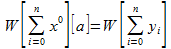Algebraically W does nothing—it simply cancels out. We can move W inside the matrices: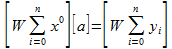And move W inside the summations: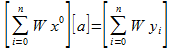Thus far we have assume W is a constant, but that was just to maneuver it into place. Now that W is in where it should be we can stop making that assumption and allow W to become a weighting term. Let W = wi so that there is a sequence of weights that can be applied to every y value. Our equation becomes: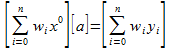We can drop the matrices, and get rid of the x0 because that is just 1.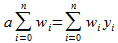Solve for a: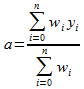This produces a weighted average with value yi weighted by wi. This matches our earlier representation of a weighted average. It is also equivalent to the mean average if the weighting term is constant, and we can quickly show this: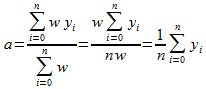We can apply the same weighting method to linear regression. We will use linear regression because the equation is not nearly as large as polynomial regression. First, the expanded representation of linear regression: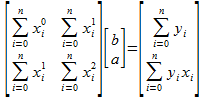Now introduce a constant weighting term: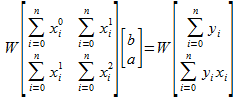Move this term into the matrix: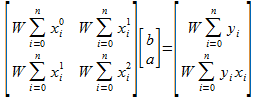And then into the summations: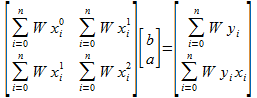Once there we no longer make the assumption that the weight value is constant, but is instead a sequence: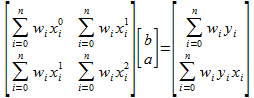Reduce the powers: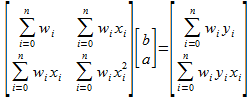And we now have the weighted form of linear regression. What was done here should be pretty straightforward—wi was just placed in all the summations. We take what we did with linear regression and can quickly apply it to polynomial regression. First start with the expanded form of polynomial regression: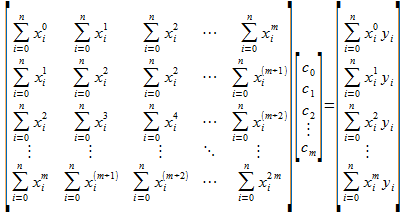Here, m is the number of coefficients for the polynomial, and n is the number of x/y data points. Now add wi was just placed in all the summations.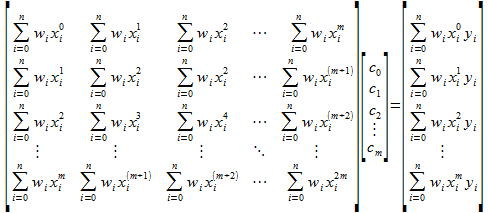Clean this up a little to simplify: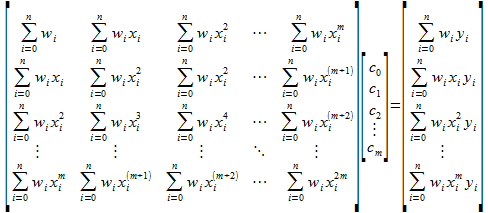This is the general form of weighted polynomial regression. Again, if the weighting term is constant for each index it will simply reduce out and one will be left with unweighted polynomial regression.

Just as with using polynomial regression, how weighting is actually used is not so easy to generalize. One must carefully consider what phenomena is being modeled before adding a weighting term to the data. However once this is understood a weighting term can be very useful.